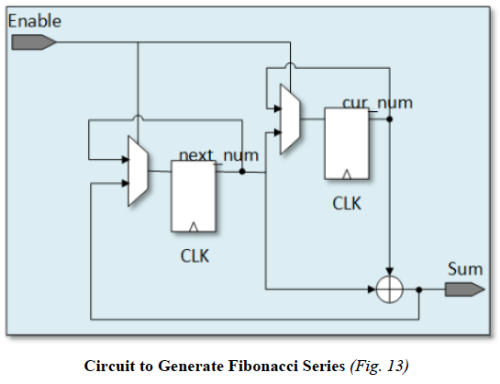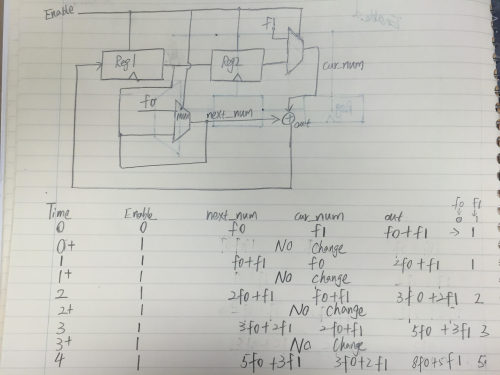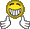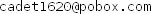# Implement Fibonacci Sequence generatorClassicListThreaded6 messagesOpen this post in threaded view
|

## Implement Fibonacci Sequence generator

 Hi, everyone, I'm thinking about how to make a fibonacci sequence generator with two Registers. While I'm working on it, I doubt if it is feasible here because I'm stuck somewhere which will be described. Here is the basic circuit I used for this purpose.The initial two inputs are two numbers f0 and f1, and they are connected to the next_num and cur_num, respectively. The first register is basically the most left mux and the flip flop and the second register is the right mux and the neighboring flip flop. Assuming my Register stores 4-bit long numbers, so, obviously, we will have this: Register4(in=sum, load=enable, out=next_num); But if I do this, what should I do with the input f0? I don't know how to set f0 to next_num as the initial value. Similarly, I'm not sure how to deal with the cur_num and the f1. Any thoughts for this?
Open this post in threaded view
|

## Re: Implement Fibonacci Sequence generator

 Administrator Implement this like PC, except that it has two Registers and no reset input. load input loads f0 and f1 inputs into the cur_num and next_num Registers. Circuit output as you have it will be f2, f3, ... If you want to see f0, f1, f2, ... use cur_num.out. It's useful to realize that Register with load=true is functionally a DFF16. --Mark
Open this post in threaded view
|

## Re: Implement Fibonacci Sequence generator

 Thanks for your quick reply, Mark, I see the approach here is much like the PC.
Open this post in threaded view
|

## Re: Implement Fibonacci Sequence generator

 In reply to this post by cadet1620 Hello, Mark, I think I get the circuit correct now as shown below but I'm not so confident about it since there is issue when I tried to load the Fibonacci.hdl file. Can you please take a look at my circuit below? The value of f0 and f1 is 0 and 1, respectively.If you want to take a look at my source files, let me know as well.I appreciate your help here.
 Administrator Your circuit as drawn has the output of Reg1 connected to the output of the f0 multiplexor. That's probably what the HardwareSimulator is complaining about. You might want to get Logisim. It's a nice schematic based logic simulator that will help you explore circuits like this.  Too bad it doesn't import or export HDL. Feel free to email your files to me and to ask questions by mail. --Mark